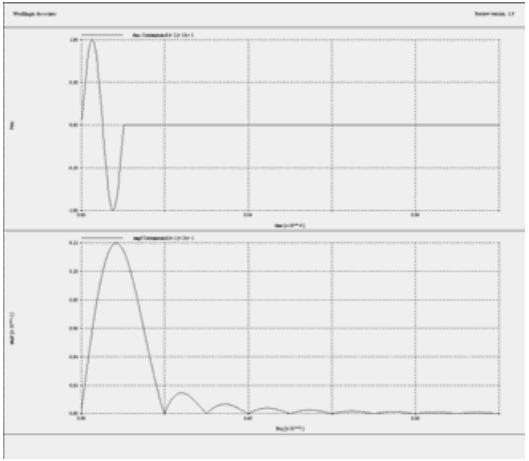# Mathematical Functions

Review allows you to mathematically manipulate the OnScale time-history data. Manipulations can range from simple scaling of the data to Fourier transformations of the time-domain data.

Most of the mathematical functions are in Review's MAKE primary command. It is common practice when manipulating previous data records to store the results in a new local file. This keeps the modified data separate from the original time history file, preventing data corruption.

Take, for example, a time history file named results.flxhst, which contains seven time histories:

• x, y, z velocity
• x, y, z velocity
• pressure

A good way to begin is by learning how to determine the difference between two time histories. The x velocity is the first array stored in 'f1 1' and the y-velocity in 'f1 2'

Read in the overall time history, create a new local file for the results, and do the calculation:

`read f1 results.flxhstmakefile f2curv { f1 1 } / { f1 2 }`

Use the READ command to enter the calculated time-history file and then the MAKE primary command. Then use the MAKE FILE subcommand to create a new local file named f2 and use MAKE CURV to subtract record 2 in file f1 from record 1 in file f1. The difference between the two files is stored in record 1 of file f2 by default.

NOTE:

1. The MAKE CURV subcommand uses curly brackets.

2. When you generate a text batch file for use in Review, the same rules govern the use of subcommands as in OnScale, i.e., the subcommand is indented in the text batch file.

The following, slightly more complex example, shows how to determine the absolute magnitude of displacement of a node with respect to its initial position. In the time history for this example, the x, y, and z displacements are stored in arrays 4, 5, and 6 respectively. Enter:

`read f1 results.flxhstmakefile f2curv sqrt ( { f1 4 } ** 2. + { f1 5 } ** 2. + { f1 6 } ** 2. )`

Take the square root of the sum of all the displacements squared. The magnitude of displacement is stored in record 1 of file f2, again by default. Note again the use of curly brackets to specify the array records.

To calculate, say, the radial velocity (in the y-z plane) of a node at 30 degrees, the "curv" subcommand is:

`curv cos ( 30. * 3.14159 / 180 ) * { f1 2 } + sin ( 30. * 3.1459 / 180 ) &* { f1 3 }`

To simplify this large input string, use the symbol or "symb" command to break up the

equation:

`symb angle = 30.symb cosval = cos ( \$angle * 3.14159 / 180 )symb sinval = sin ( \$angle * 3.14159 / 180 )curv \$cosval * { f1 2 } + \$sinval * { f1 3 }`

To save the data in the new local file f2, exit the MAKE command directory and write the local file to an external file:

`writ f2 newfile.dat`

Here the local file f2 is written to a file newfile.dat.

Converting to Frequency Domain

You can also use Fourier transform techniques to translate the data in the time-history files from the time domain to frequency domain. This is done not with the "make" command, but with the frequency input or "freq" command.

For a time-history file with the drive input function of a single-cycle sinusoid at 1MHz in the first array, you can use the "freq" command and associated subcommands to convert it to the frequency domain:

`read f1 results.flxhstfreqfile f2fft f1 1endgrphnvew 2 1plot f1 1plot f2 1end`The second graph shows the frequency response corresponding to the FFT of the time-domain signal in the first graph. Any single FREQ command creates two frequency domain records: amplitude and phase by default, or, if requested with the FREQ TYPE subcommand, real and imaginary.

0 out of 0 found this helpful